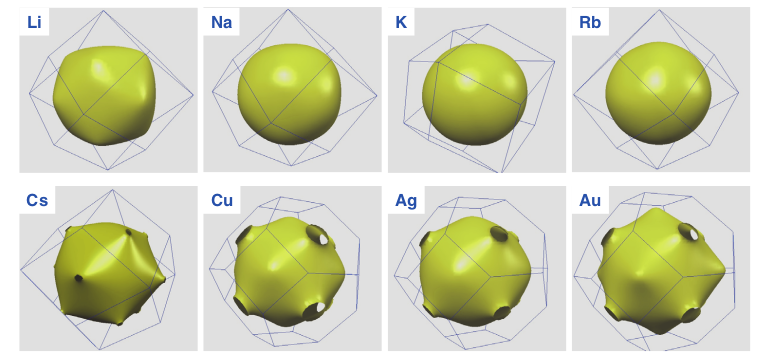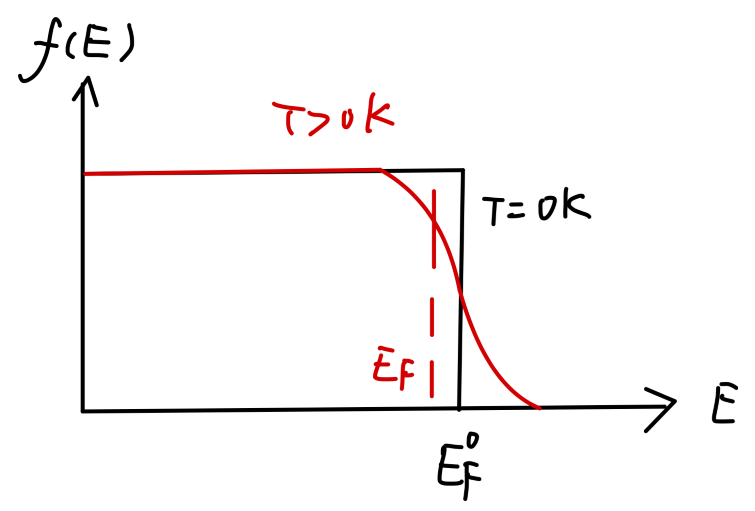## 费米统计与电子比热

### 基态的费米分布函数和费米能

$f(E) = \frac{1}{1 + e^{(E - E_F)/k_BT}} \tag{1}$

$E_F$ 为化学势，对于费米子系统来说，称为 费米能

$\int_{0}^{\infty} f(E)N(E)dE = N \tag{2}$

$E = E_F$ 时，得到：

$f(E_F) = \frac{1}{2}$

$f^0(E) = H(E_F^0-E) \tag{3}$

$-\frac{\partial f^0}{\partial E} = \delta(E-E_F^0) \tag{4}$

$\frac{\hbar^2}{2m}{k_F^{0}}^{2} = E_F^0 \tag{5}$Fig：碱金属的费米面

\begin{aligned} \int_{0}^{\infty}f^0(E)N(E)dE = \int_{0}^{E_F^0} \frac{2V}{(2\pi)^2}(\frac{2m}{\hbar})^{3/2}E^{1/2}dE =N \end{aligned}

\begin{aligned} E_F^0 &= \frac{\hbar^2}{2m}(\frac{3\pi^2 N}{V})^{2/3}\\ & = \frac{\hbar^2}{2m} (3\pi^2n_0)^{2/3},\quad n_0 = \frac{N}{V}\\ \end{aligned} \tag{6}

\begin{aligned} &E_F^0 \sim 1.5\sim 7 \mathrm{eV}\\ &k_F^0 \sim 10^8 \mathrm{cm}^{-1}\\ \end{aligned}

$mv_F = \hbar k_F^0 \tag{7}$

\begin{aligned} U_0 &= \int_{0}^{\infty} E f^0(E) N(E)dE \\ &= \int_{0}^{E_F} \frac{V}{2\pi^2}(\frac{2m}{\hbar^2})^{3/2} E^{3/2} dE\\ &= \frac{2}{5}\frac{V}{2\pi^2}(\frac{2m}{\hbar^2}) {E_{F}^{0}}^{5/2}\\ & = \frac{3}{5}NE_F^0 \end{aligned}

$\frac{U_0}{N} = \frac{3}{5}E_F^0 \tag{8}$

\begin{aligned} B &= \frac{1}{\kappa} = -V \frac{\partial p}{\partial V} = V^2 \frac{\partial^2 U_0}{\partial V^2}\\ & = \frac{2}{3}n_0E_F^0\\ \end{aligned} \tag{9}

### 激发态的分布函数和费米能Fig：基态和激发态的分布函数

$T = 0, E_F^0 \sim 5 \mathrm{eV}$

$k_BT = E_F^0 \Rightarrow T_F = \frac{E_F^0}{k_B} \sim 5\times 10^4 \mathrm{K}$

$T/T_F \sim 10^{-2}$

$f(E) = \frac{1}{1 + e^{(E - E_F)/k_BT}}$

$-\frac{\partial f}{\partial E} = \frac{1}{k_BT} \frac{1}{(e^{(E-E_F)/k_BT} + 1)(e^{-(E-E_F)/k_BT} + 1)}$

f(E) = \frac{1}{e^{(E-E_F)/k_BT}-1} \approx \left\{ \begin{aligned} & 1 & E-E_F \ll -k_BT\\ & \frac{1}{2} & E= E_F\\ & 0 & E-E_F \gg k_BT\\ \end{aligned} \right.

$-\frac{\partial f}{\partial E} \approx \delta(E-E_F)$

$N = \int_{0}^{\infty}f(E)N(E)dE$

\begin{aligned} &Q(E) = \int_{0}^{E} N(E)dE\\ &Q'(E) = N(E)\\ \end{aligned}

\begin{aligned} N =& \int_{0}^{\infty} f(E)Q'(E)dE\\ =& f(E)Q(E)|_{0}^{\infty} + \int_{0}^{\infty} Q(E) (-\frac{\partial f}{\partial E}) dE\\ =& \int_{0}^{\infty} Q(E) (-\frac{\partial f}{\partial E}) dE\\ =& Q(E_F) \int_{-\infty}^{\infty} (-\frac{\partial f}{\partial E})dE + Q'(E_F) \int_{-\infty}^{\infty} (E-E_F)(-\frac{\partial f}{\partial E}) dE\\ &+ \frac{1}{2}Q''(E_F) \int_{-\infty}^{\infty} (E-E_F)^2 (-\frac{\partial f}{\partial E})dE + \cdots \end{aligned}

$N = Q(E_F) + \frac{1}{2}Q''(E_F) \int_{-\infty}^{\infty} (E-E_F)^2(-\frac{\partial f}{\partial E}) dE + \cdots$

$\xi = \frac{E-E_F}{k_BT}$，那么可以从上式得到：

\begin{aligned} \int_{-\infty}^{\infty} (E-E_F)^2 (-\frac{\partial f}{\partial E})dE &= (k_BT)^2 \int_{-\infty}^{\infty} \frac{\xi^2d\xi}{(e^{\xi}+1)(e^{-\xi}+1)}\\ &= \frac{\pi^2}{3}(k_BT)^2\\ \end{aligned}

\begin{aligned} N &= Q(E_F) + \frac{\pi^2}{6} Q''(E_F)(k_B T)^2\\ &= Q(E_F^0) + Q'(E_F^0)(E_F-E_F^0) + \frac{\pi^2}{6} Q''(E_F^0)(k_B T)^2\\ &= N + Q'(E_F^0)(E_F-E_F^0) + \frac{\pi^2}{6} Q''(E_F^0)(k_B T)^2\\ \end{aligned}\tag{10}

$E_F = E_F^0 - \frac{\pi^2}{6}\frac{Q''(E_F^0)}{Q'(E_F^0)}(k_BT)^2 \tag{11}$

$E_F = E_F^0\{1 - \frac{\pi^2}{12}(\frac{k_BT}{E_F^0})^2\} \tag{12}$

### 电子热容量

\begin{aligned} U &= \int_{0}^{\infty} Ef(E) N(E)dE\\ \end{aligned}

\begin{aligned} U(E) &= R(E_F^0) + (E_F - E_F^0)R'(E_F^0) + \frac{\pi^2}{6}R''(E_F^0)(k_BT)^2 \end{aligned}

\begin{aligned} &R(E_F^0) = U_0\\ & E_F - E_F^0 = - \frac{\pi^2}{12E_F}(k_BT)^2\\ & R'(E_F^0) = E_F^0 N(E_F^0)\\ & R''(E_F^0) = N(E_F^0) + E_F^0N'(E_F^0)\\ \end{aligned}

$U = U_0 + \frac{\pi^2}{6} N(E_F^0)(k_BT)^2 \tag{13}$

\begin{aligned} C_V &= (\frac{\partial U}{\partial T})|_V \\ &= \frac{\pi^2}{3} N(E_F^0)k_BT \cdot k_B\\ &= N\frac{\pi^2}{2} (\frac{k_BT}{E_F^0}) k_B\\ & = \frac{\pi^2}{2}N k_B (\frac{k_BT}{E_F^0})\\ & = \frac{\pi^2}{2}N k_B (\frac{T}{T_F^0})\\ \end{aligned}\tag{14}

$C_{V} = \gamma T + b T^3 \tag{15}$

1. 黄昆 固体物理学
2. 胡安 固体物理学